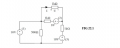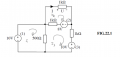# Mesh current analysis help

#### hBryne

Joined Nov 18, 2020
6
Hi!

I have a small circuit that's giving me a headache for one of my lab assignments. I've gone through it with node voltage analysis for the previous lab with confirmed results, but I'm stuck on completing the mesh current analysis of the same circuit.Pasted the circuit above. We need to determine the current I and voltage U. They should be I = 2.67mA and U = 2.67V.

I think I've gotten the mesh equations right, with I1(left) = 41mA, I2(top) = 15mA and I3(corner) = 21mA
but I don't really know where to go from here to get the right result up top.

Would very much appreciate pointers//Bryne

#### ericgibbs

Joined Jan 29, 2010
18,211
hi Bryne,
Welcome to AAC.
E

#### hBryne

Joined Nov 18, 2020
6
Hi Eric,

$I_1: 10V-500\Omega (I_1-I_3) = 0$
$I_2: 8V-1000\Omega (I_2-I_3) - 1000\Omega(I_2) = 0$
$I_3: -10V + 500\Omega (I_3-I_1) + 1000\Omega (I_3-I_2) - 8V - 1000\Omega (I_3) = 0$

Plugged them into Microsoft Mathematics to solve for the answers I got

#### hBryne

Joined Nov 18, 2020
6
I guess what i'm really asking is how to calculate the current and voltage in the top branch with this method.
With this covid lockdown we're pretty much fending for ourselves with bad textbooks..

With node voltage analysis I calculated the voltage at the right hand node and then just subtract for voltage drop over the top resistor.

In mesh analysis I'm not sure how to proceed.

#### WBahn

Joined Mar 31, 2012
29,469
The problem is that your mesh equations are not correct.

It's important to realize that all of the physics (i.e., the "EE stuff") is captured by those equations. Everything after that is just math. If those equations aren't right, everything that follows is wasted effort. Worse, it is very difficult to catch errors after that because you are simply solving a different problem.

So get in the habit of getting your setup equations in place and then, before you do anything else, vet them carefully. Go through term by term asking if each part of it is correct, including the signs.

Do that and you should find your two errors.

#### hBryne

Joined Nov 18, 2020
6
The problem is that your mesh equations are not correct.

It's important to realize that all of the physics (i.e., the "EE stuff") is captured by those equations. Everything after that is just math. If those equations aren't right, everything that follows is wasted effort. Worse, it is very difficult to catch errors after that because you are simply solving a different problem.

So get in the habit of getting your setup equations in place and then, before you do anything else, vet them carefully. Go through term by term asking if each part of it is correct, including the signs.

Do that and you should find your two errors.

Thanks alot to both of you, I'll have a look from the beginning again. Seems I chose the wrong place to think myself stuck//Bryne

#### hBryne

Joined Nov 18, 2020
6
Well. Went through and got the correct values instantly.
I think I got confused in taking the differences between meshes over shared components..

Thank you both again!

#### ericgibbs

Joined Jan 29, 2010
18,211
hi Bryne,
Please post your calculations, other students may benefit from your work.E

#### hBryne

Joined Nov 18, 2020
6
Yes, good idea Eric.

So, problem: Find the current I and voltage U using mesh current analysis.

Defined mesh I1-3 according to numbering on the voltage sources with clockwise direction.Then I derived functions for each mesh, going negative for voltage rise and positive for voltage drop:

This is where I went wrong, with the negatives and positives.

$$I_1: -10V + 500\Omega (I_1-I3_) = 0$$
$$I_2: -8V + 1000\Omega (I_2-I_3) + 1000\Omega(I_2) = 0$$
$$I_3: 500\Omega (I_3-I_1) + 1000\Omega (I_3-I_2) + 8V + 1000\Omega (I_3) + 10V = 0$$

I took the equations in to microsoft mathematics to solve but not sure if that saved any time.

$$I_1 = 17.33 mA$$
$$I_2= 2.67 mA$$
$$I_3= -2.67 mA$$

$$I = I_2 = 2.67mA$$

$$U = RI = 1000 \times 0.00267 = 2.67V$$

And that was that - didn't really need to solve for I1 and I3 here but it's all for learning anyways.

I am sure there still are issues here but the next few lab assignments for mesh current analysis went well atleast, consistent with measurements and spice.

//Bryne

•ericgibbs

#### WBahn

Joined Mar 31, 2012
29,469
Ah, you were doing so well....

You included the units in your set up equations, but then dumped them in that last step. It should have been

U = R·I = 1000 Ω · 0.00267 A = 2.67 V

Always track your units properly throughout your work. It will let you catch most mistakes you make (and we all make them) right when you do it.

•hBryne